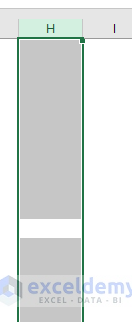# How to apply same formula to multiple cells in Excel (8 easy ways)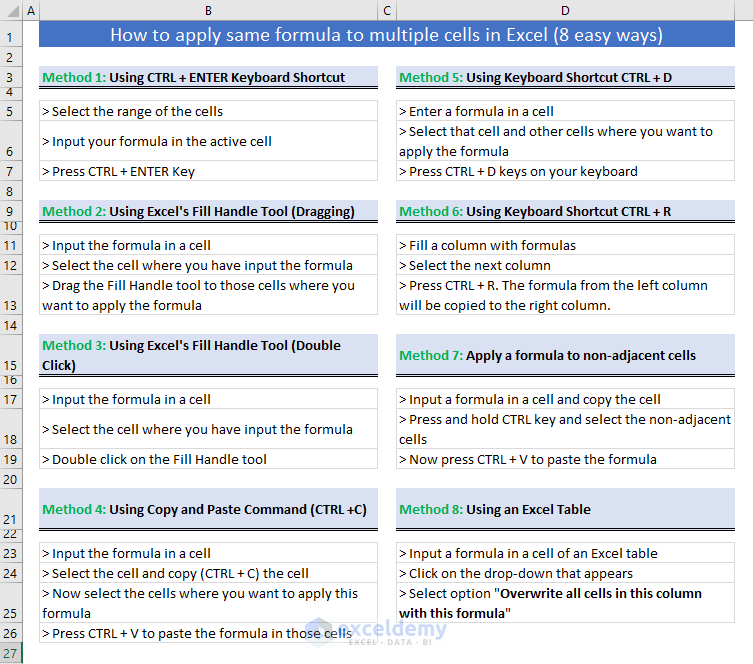In this tutorial, I will show you how to apply same formula to multiple cells in Excel.

When we will do so, cell references will also change according to the types of references we have used in the formula.

Let’s learn the methods.

At first, download the working file that I have used to make this tutorial.

## Applying the same Excel formula to multiple cells (cell references will change)

In more than one way, you can apply an Excel formula to multiple cells (cell references will also change). Let’s check out them. Use the method that best suits your purpose.

### 1) Using CTRL + Enter Keyboard Shortcut

You are seeing a sample data in the picture below. The prices of some products (in Euro) are given under the Price (Euro) column. Also, the exchange rates are shown in the cell range C2:E2. What I want is to show the prices of the products in other currencies such as USD, GBP, and JPY.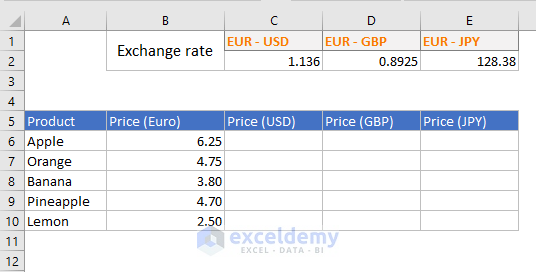I will write just one formula in the cell C6 and will use it over cell range C6:E10.

Let me show you how to do it.

Step 1

Select the cell range C6:E10.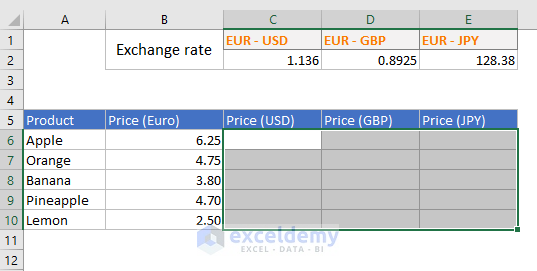When you select a range of cells, the first cell becomes the active cell. For our selection, cell C6 is the active cell. You see the other cells in the range are in grey color (not active).

Step 2

Now type an equal sign and write this formula: =\$B6*C\$2. This formula will be automatically entered into the cell C6 as C6 is the active cell.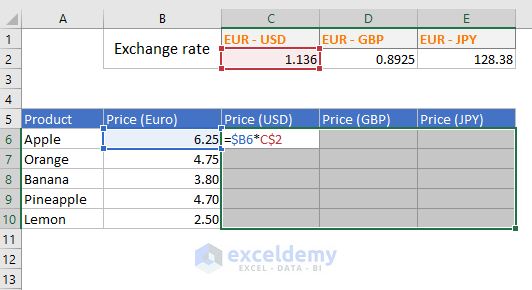Step 3

Press CTRL + Enter simultaneously on your keyboard. This is what you will get.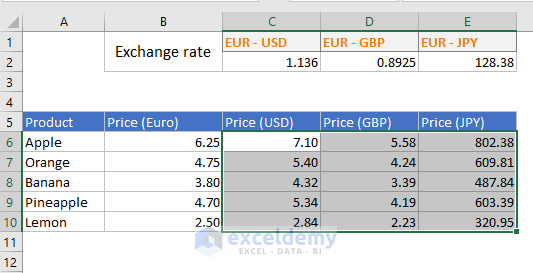How does this formula (=\$B6*C\$2) work?

• Notice that the formula has mixed cell references. \$B6 reference means that when the formula will be copied to the right, column B will not change. So, it will be like B6, B6, and B6. All are B6 as when the formula is copied to right, the row is not changing. When the formula will be copied down, the cell reference will be B6, B7, B8, B9, B10. This is because the rows change when you copy down a formula.
• C\$2 reference means that when the formula will be copied to the right, the references will be C2, D2, and E2. Because the reference of column C is relative. And when we shall copy this formula down, the references will be C2, C2, C2, C2, and C2. This is because row 2 is absolute.
• Let’s check a cell in the range. I select cell reference D8 and press F2 key (this takes the cell to Edit mode) on the keyboard. The formula is working perfectly.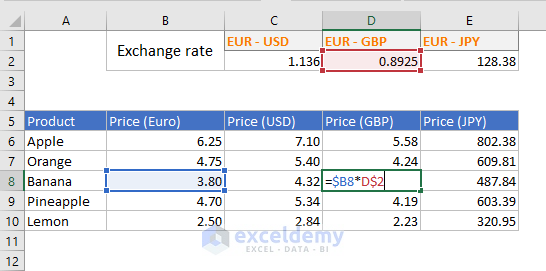### 2) Using Excel Fill Handle Tool (Dragging)

I will use the same example to explain this method. This time, I will select only the cell C6 and enter this formula: =\$B6*C\$2. Then press Enter key. We shall get value 7.10 in the cell C6. Again, select the cell C6.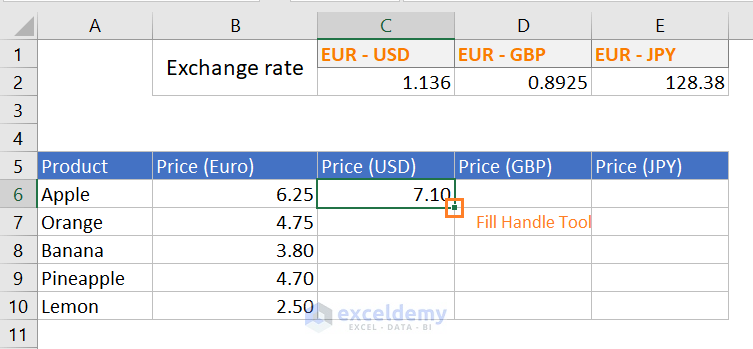Observe the above image. You will see a green color solid square at the bottom right corner of the cell. It is the Fill Handle tool in Excel.

• Move your mouse pointer over the Fill Handle solid. The big white plus sign will turn into a small black sign
• Click and hold your mouse pointer and drag down. Then using the same method, drag to the right.
• This is the complete method shown in the gif image.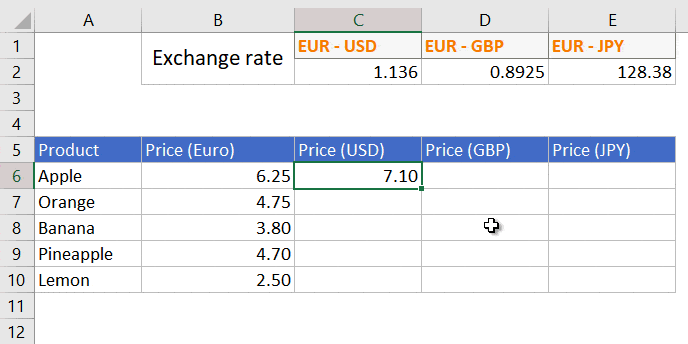I will not explain how the formula works because it is already done in the above method.

### 3) Using Excel Fill Handle Tool (Double click)

You can also double-click on the Fill Handle tool. The formula will be copied to the cells below (up to the cell where adjacent column data is available).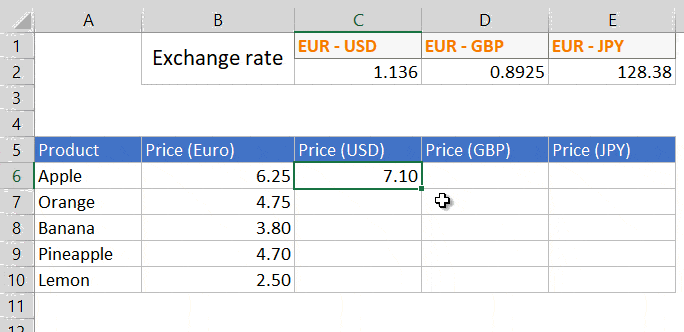Note: You cannot use this method to apply a formula to the cells on the right.

What to do when the Fill Handle tool does not show?

Is it the case that Fill Handle tool appears at the cells but it is not working? Then it is not activated. Follow these steps to activate it:

• File tab > Options
• Excel Options dialog box appears > click on the Advanced
• Under Editing Options > check the Enable fill handle and cell drag-and-drop box
• Finally, click on the OK You’re all set.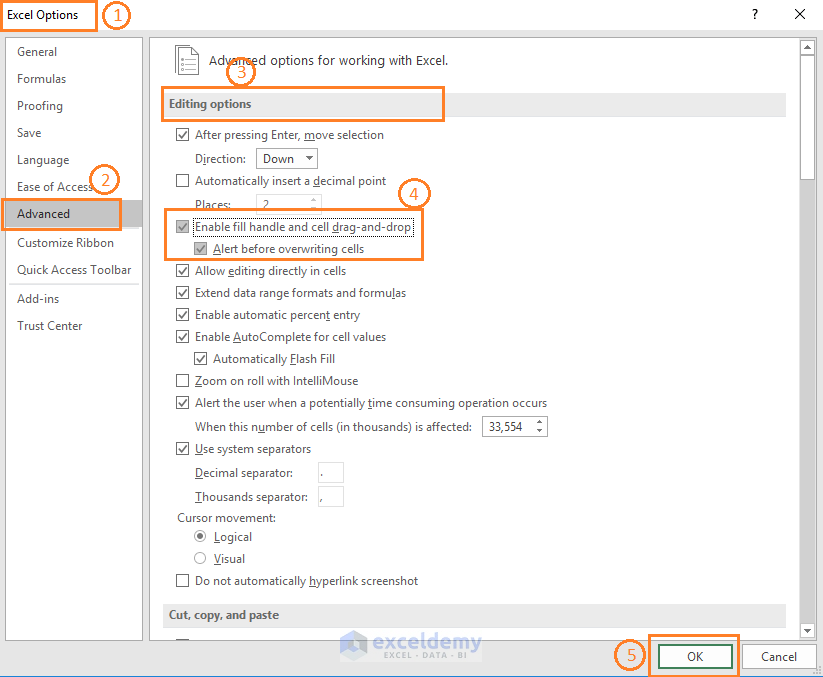### 4) Using Copy and Paste Command (CTRL + C)

• Select the cell C6 (=\$B6*C\$2) and copy it using CTRL + C keyboard shortcut or using the command button in the Home > Clipboard group of commands.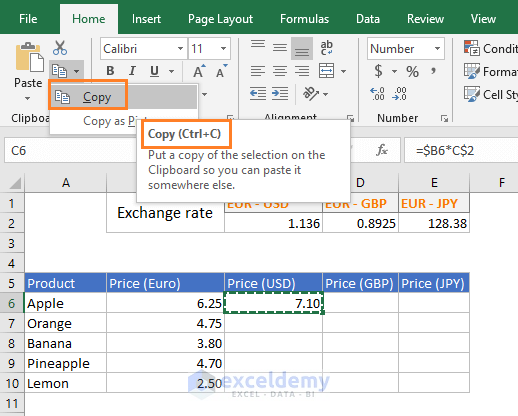• Now select the cell range C6:E10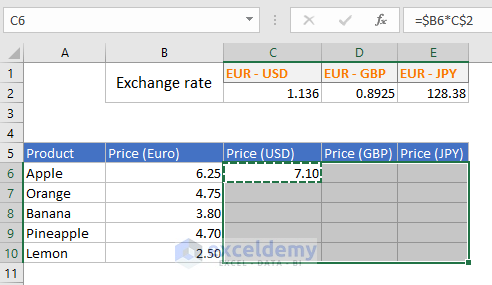• Then use the Home > Clipboard > Paste command or press CTRL + V keyboard shortcut.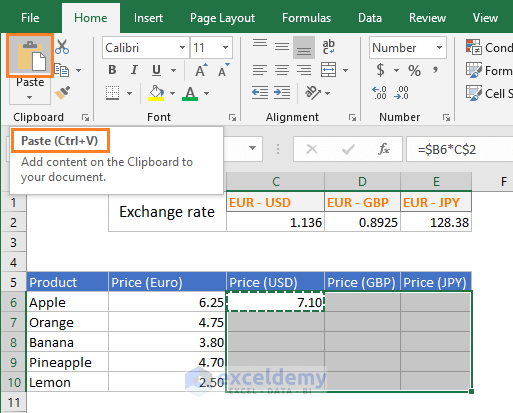• This is what we get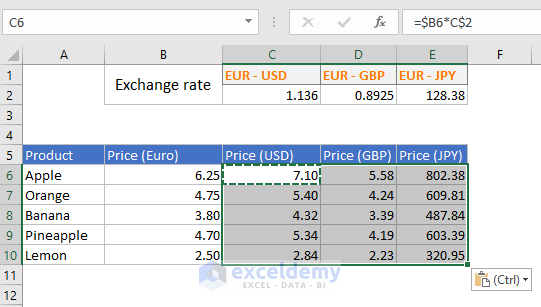### 5) Using Keyboard Shortcut CTRL + D

• Place this Excel formula in the cell C6: =\$B6*C\$2 and press Enter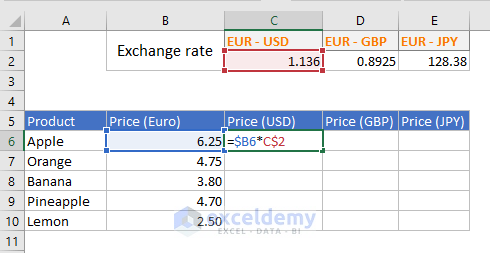• Now select cell C6 and the other cells in the column where you want to apply the formula.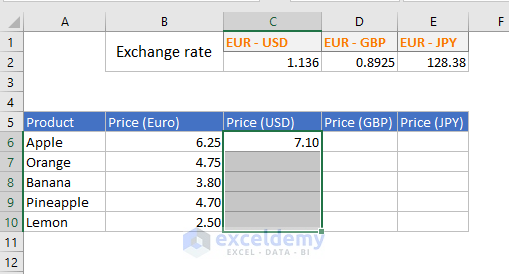• Now press CTRL + D simultaneously on your keyboard. The whole column is filled with the formula in the cell C6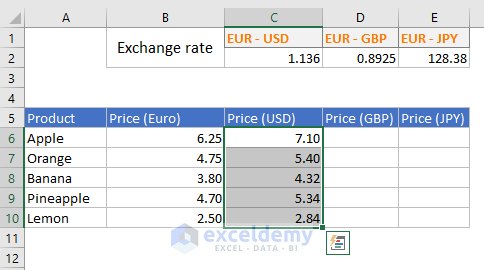Note: Using CTRL + D keyboard shortcut, you cannot apply a formula to a range. It works only for the column.

### 6) CTRL + R keyboard shortcut to apply formula on the column to the right

Suppose you have made a column filled with Excel formulas like the following image.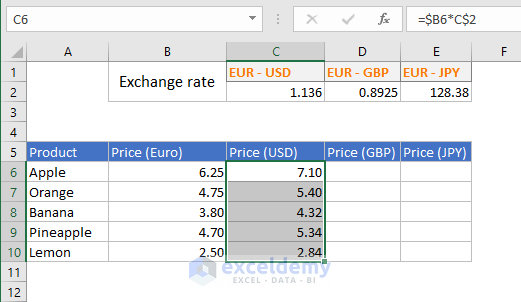Now you want to apply this formula set to the right column. To do this, you can use the CTRL + R keyboard shortcut.

• Select the right column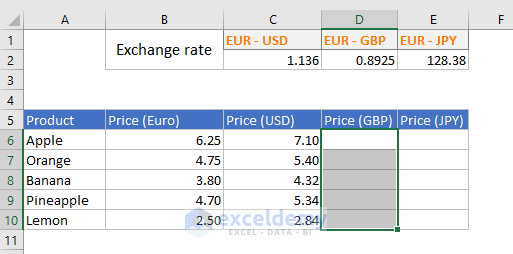• Now press CTRL + R keys on your keyboard. This is the result.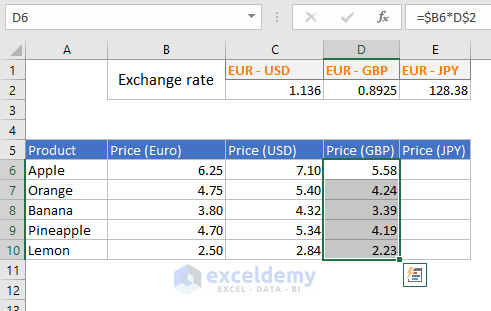Note: You cannot use apply this shortcut in more than one column.

### 7) Copying a formula to multiple cells but the cells are non-adjacent

To apply a formula to non-adjacent multiple cells, you can use these two methods:

• Using CTRL + C keyboard shortcut
• Or using CTRL + D keyboard shortcut

# Using CTRL + C keyboard shortcut

• Copy the formula in the cell C6
• Press and hold the CTRL key on your keyboard and then select all the cells where you want to apply the formula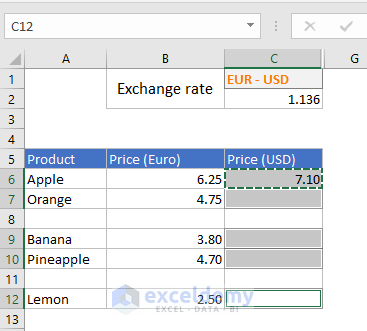• Now press CTRL + V on your keyboard. You’re done.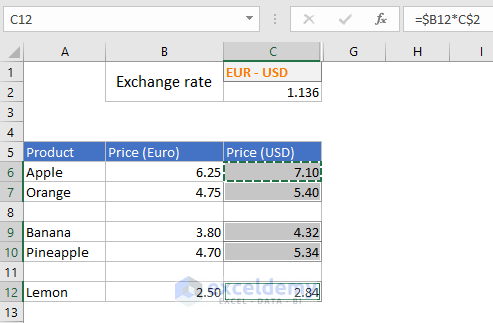# Using CTRL + D Keyboard Shortcut

• Select the cell (C6) where the formula is and all the other cells where you want to apply the formula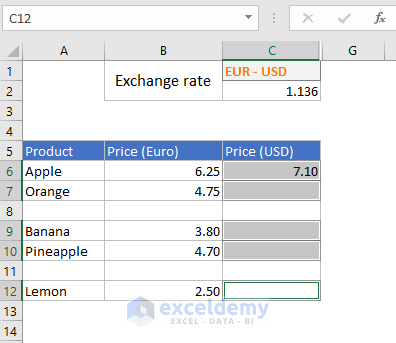• Now press CTRL + D on your keyboard. You’re done.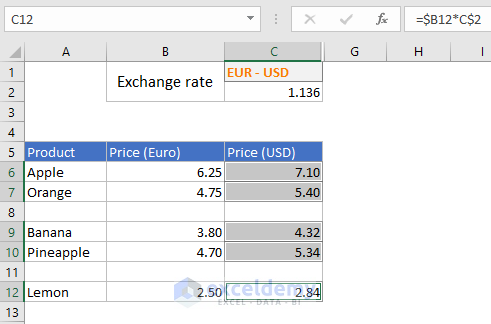### 8) Using an Excel Table

We have a dataset like shown in the image below. The data is set in an Excel table. Sometimes I think, an Excel table is one of the wonders of our civilization.

It is easy to convert a range to a table. Just select a cell within the range and press CTRL + T or use the command Insert > Tables > Table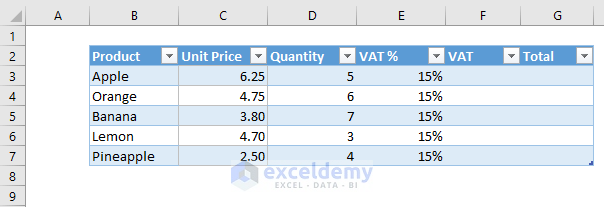Let’s calculate the VAT for the items for these products.

• Select cell reference F3 > Input an equal sign > Select the first cell of the Unit Price column > Multiplication symbol (*) > Select the first cell of the Quantity column > Multiplication symbol (*) > Finally select the first cell of the VAT % column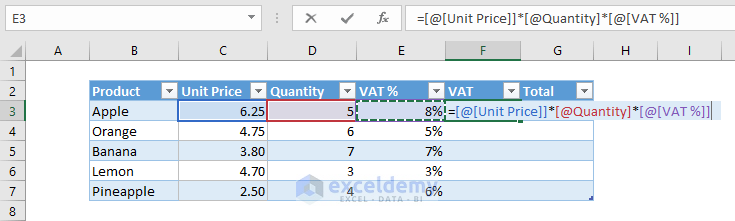• Press Enter key > VAT will be calculated > A drop down will appear > Click the drop-down and select the option Overwrite all cells in this column with this formula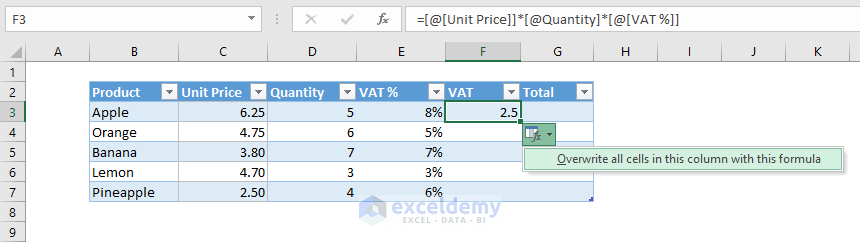• This is what we get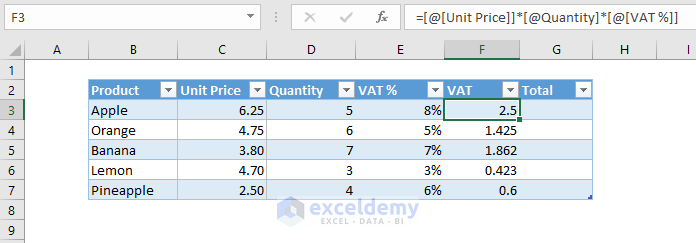When we use a formula in Excel table, the references are actually structured references, not regular cell references.

To use regular references in an Excel table formula, you have to type the references manually: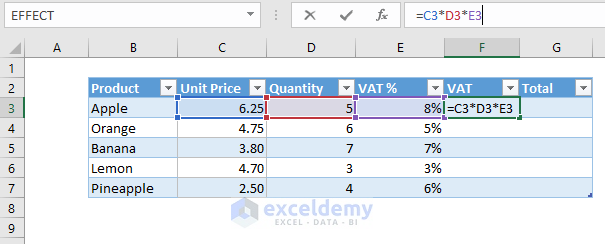## Learn some important keyboard shortcuts

### 1) CTRL + SHIFT + END

To select the whole range after inserting the formula in the cell C6, press CTRL + SHIFT + END key on your keyboard.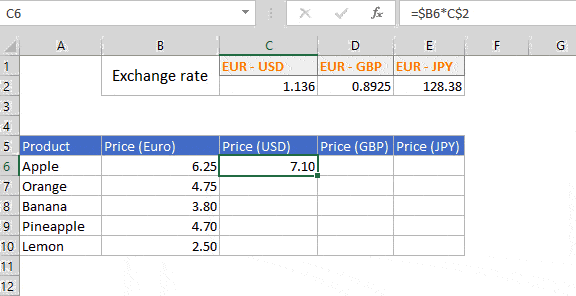### 2) CTRL + SHIFT + DOWN ARROW to select the column data from the cell where you are

This keyboard short CTRL + SHIFT + DOWN ARROW will select the column up to the cell where data is available. Further pressing this shortcut will select the whole from your location.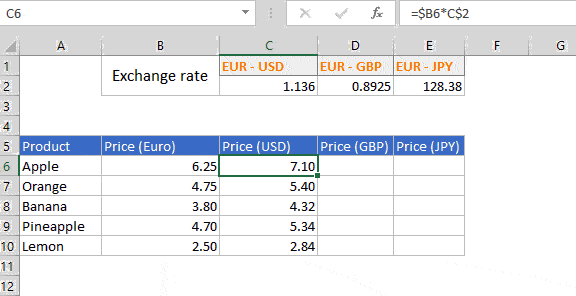### 3) CTRL + SPACE will select the whole column